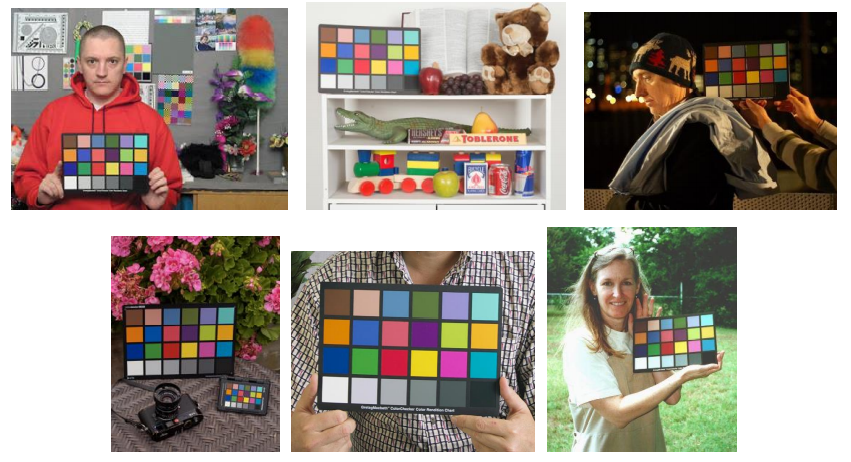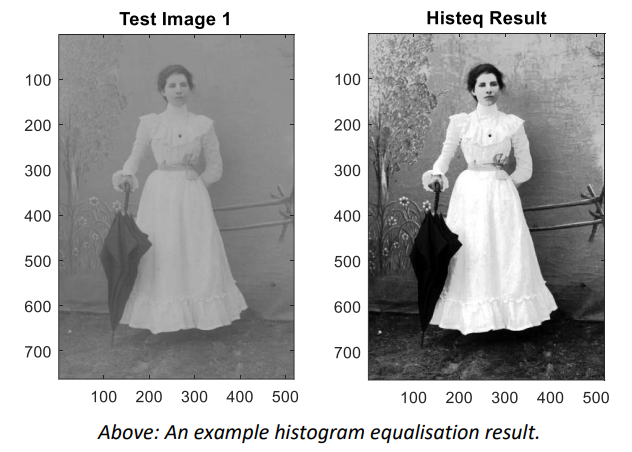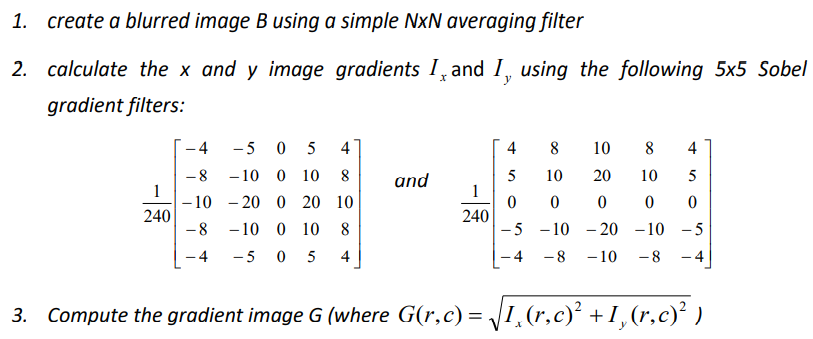Search

# MATLAB Homework Help | Image Processing Using MATLAB-Sample Paper

Overview

This assignment is intended to give you some hands-on experience with manipulating image data in MATLAB and the basic image manipulation concepts covered in the course up to the end of Week 3.

In this assignment work you will be required to modify several functions which implement basic colour correction, contrast enhancement and noise filtering steps.

Assignment Submission

Please see MyUni for details on the due date (approximately late August).

Assignments are to be submitted as a standard ZIP archive file (please do NOT use other formats such as 7z, RAR, PKZIP) containing the key MATLAB functions and any test code you have written and a Word (MS Office) or PDF document summarising your results with comments on the performance of the processing steps employed (in 1A-1D) and written answers to questions (1E)

• All submitted materials MUST be your own individual original work.

• Marks will be deducted for late submission or incorrect submission format.

Source Materials

Source code, test functions and example imagery for this assignment is located on the MyUni website (https://www.myuni.adelaide.edu.au). You are welcome to add your own example imagery for testing and reporting purposes.

Task: 1 Colour Balancing using a Reference Chart

Your company is developing a simple enhancement package to be bundled in with its new range of digital cameras. You have been tasked to quickly develop some of the processing steps which will be used in this package. The first is a tool to be used in conjunction with a colour chart to enable people to correct their photographs for the current lighting conditions.

Colour charts such as the one shown below are used by some professional photographers to allow them to colour correct their images after a photographic shoot. The colour chart is photographed under the same lighting conditions and then compared to the original chart.The differences between the true colour chart and the captured image of the colour chart are used to adjust and therefore correct the colour in subsequent images.

In this exercise you will be required to write three simple functions, the first extracts samples from a 24-colour chart image similar to that shown in the above left. The second function takes two sets of extracted values, one from the true chart and once from a chart collected under less than ideal conditions and calculates the mapping of RGB colours from the less than ideal conditions back to the ideal case. The third function applies this mapping to an image to correct for the colour imbalance.

STEP 1: Modify the supplied function get_chart_values.m so that given an image of a colour chart such as that shown above left it returns an 24x3 array of the 24 RGB values corresponding to each patch. The order in which you extract these values is not important.

eg:

```I = imread(‘color_testchart.jpg’);
values = get_chart_values(I); ```

The supplied image is assumed to contain values in the ranges 0..255, with R,G and B being the first second and third layers of the image respectively.

STEP 2: Modify the supplied function chart_correction.m so that given a set of colour chart values from a correct (reference) chart and a set of measurements from a chart captured at a photographic shoot it returns a 256x3 array containing the R,G,B colour corrections required to convert (colour correct) any pixel in an image captured under similar conditions back ‘ideal’ conditions. Row 1 of this 256x3 array contains the adjustments for red, green and blue pixels of value 0 (zero), and row 256 contains the adjustments for red, green and blue for pixels of value 255. eg.

```chart_image = imread(‘testchart.jpg’);
goodvalues = get_chart_values(chart_image);

The two colourcharts contain 24 measurements of how each of the red, green and blue image layers differ between the reference and the photo-shoot. These can be used to estimate the correction required for each red, green or blue sample from 0..255.

To calculate this, you can use existing MATLAB functions such as polyfit and polyval if you like to fit the data and estimate the missing values (recommended). Alternatively, you can set up the problem in the form Ax=b and use “\” to find the least squares solution but this takes a little more coding (not recommended). You could also use interp1 but be aware you may need to handle cases of duplicate samples (see unique).

Whichever solution you attempt make sure you enforce the resulting mapping to be integers within the ranges 0..255.

Please refer to the MATLAB documentation (eg ‘help polyfit’) for information on how to use the suggested functions.

STEP 3: Modify the supplied function apply_rgb_map.m so that given an 8-bit RGB image and a (256x3) mapping constructed by chart_correction.m it adjusts each RGB value in the map based on the map values. eg.

```…as before…

Note that the resulting image must only contain values in the range 0..255

STEP 4: Test your code using the function chart_test.m. Try other variations in colour differences and other imagery. Compare the before and after imagery and note where there are large discrepancies between the ‘ideal’ and ‘corrected’ image values.

STEP 4: Test your code using the function chart_test.m. Try other variations in colour differences and other imagery. Compare the before and after imagery and note where there are large discrepancies between the ‘ideal’ and ‘corrected’ image values.

Optionally, I have also included a final test function chart_test_advanced.m which attempts to do a proper correction for a given image by getting you to mark the location of the chart in the “bad” image. For this additional test the output imagery and may look different (better even!) than the original test card images.

An example result produced by chart_test.m is shown on the next page

GENERAL NOTE: the images used here are 8-bit unsigned integers. If required you can convert between this and type double using the conversion functions double() and uint8(). You may need to do this at various times throughout the assignment work.Above: A simulated example of applying the colour corrections functions. The results on the right are based on the comparison of the reference and ‘bad’ cards shown above. Note that in practice there is normally no reference image (lower left) and the bad test card samples (top middle) are usually taken from the bad picture (lower middle). Special

Comment: If you think you are colour blind (or there's chance you may be) then it might be a good idea to get someone else to look over your image results just in case. If in doubt, then ask me to take a look.

For this exercise, there are multiple images available for testing and experimenting (or you can even find your own off of the internet) …Task : 2 Image Contrast Enhancement

STEP 1: Modify the function histeq_contrast.m and implement the histogram equalisation steps described in class. You may use the MATLAB inbuilt functions hist(), sum(), cumsum(), max() and min() (or even histcounts() in later versions) to achieve this. For clarity, the equation shown in notes can be written as:The call for this function is simply:

`eq_image = histeq_contrast(input_image);  `

The input image data is assumed to be in the range 0.0 to 1.0. It is recommended you use a histogram with 256 levels. This will allow you to create m() as a 256 entry lookup table using the above expression. Values in this table can then be used to replace the original image values by multiplying the image value by 255 and adding 1 (which provides the index into the lookup table).

An example result for histogram equalisation is shown at the bottom of this page. A simple test function histeq_test.m has been supplied to help you check your code.STEP 2: write up your results and include example imagery before and after processing using the equalisation steps (use other images as well as the test example shown above).

Comment: you can use the function hist() (or histcounts() if you are using a more recent version of MATLAB) in your solution but make sure you read the help information carefully. Do NOT use imhist() etc.

Task 3: A Simple median Filter

STEP 1: Modify the function median_filter.m to implement a simple MxN median filter. You may not use functions such as median(), ordfilt2(), medfilt1(), medfilt2() etc but you may use sort().

The function median_filter.m is called as follows:

`med_image = median_filter(input_image,M,N); `

If the MxN neighbourhood contains an odd number of samples the median is simply the middle value of the sorted list of neighbouring samples. However, if MxN is even the median is the average of the values to either side of the midpoint (eg. the median of [ 1 2 3 4 5] is 3 and the median of [1 2 3 4] is 2.5).

STEP 2: Use the median_test.m function to test your solution. You may use medfilt2() as a check of your output but be aware that you may handle pixels near the image boundary differently to how the inbuilt function does.

Try applying your solution to various imagery. As with median_test.m you may use imnoise() to add salt and pepper noise to imagery of your choosing.

Try increasing the proportion of salt and pepper samples and see what happens.Write up your results including example imagery (some samples have been provided). Quick question: Under what noise conditions does the median filter fail?

Task 4: A "Smart" Edge Preserving Noise Filter

To reduce the visible effects of image-sensor noise your company has been forced to to implement an averaging filter step. However, as applying an average (blurring) filter to the entire image will also blur details such as edges you have been tasked to implement and test a simple "smart" solution which attempts to preserve edge details where possible. This is achieved by reducing the proportion of the averaged image returned by the filter in areas of the image where the image gradients are large.Above: An example of applying a simple edge preserving averaging filter to a portion of a noisy image containing sharp details. The input image (top left) is shown after blurring (top right). The gradient based weights used to combine the blurred and original images are shown (bottom left) with the final "smart" blurred image (bottom right). Note the preservation of details in the hard edges of the structure.

The proposed smart filter process is as follows:STEP 1: Implement the above algorithm in the supplied function smart_blur.m. You may use matlab functions such as conv2(), and min() to simplify your solutions. Speed is not a concern in your solution.

Note that the function smart_blur.m takes 3 parameters and returns an image result. That is:

`new_image = smart_blur(noisy_image,N,tolerance); `

Here N specifies the size of the NxN averaging filter to apply and tolerance is the value t used in the above expressions.

STEP2: Test your solutions using the script smart_blur_test.m and then experiment with different images and values for the key parameters N and tolerance.

The matlab function imnoise() can be used to add noise to any image if required (however I suggest you initially used modest levels of noise).